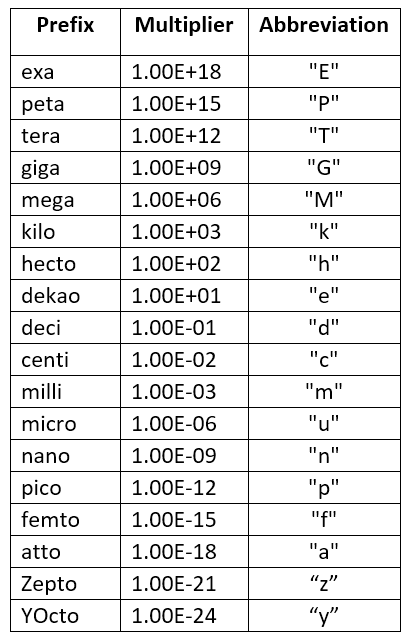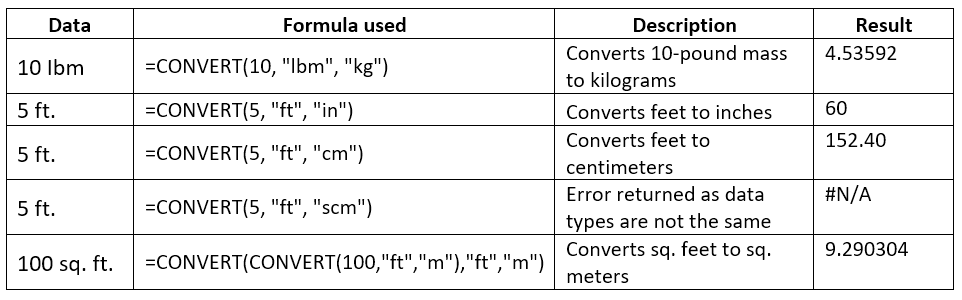# CONVERT Function

Converts a number from one measurement system to another

## What is the Excel CONVERT units Function?

The Excel CONVERT Function is an Excel Engineering Function. The function is useful for conversion of units from one measurement system to another measurement system. For example, CONVERT will help us to convert pounds to kilograms, feet to centimeters, or Fahrenheit to Celsius. Learn Excel unit conversion in this guide.

### Formula

=CONVERT(number, “from unit “,”to unit”)

The Excel CONVERT function uses the following arguments:

1. Number (required argument) – The numeric value we wish to convert.
2. From Unit – This is the unit you are converting from. Quotations are necessary!
3. To Unit – This is the unit you are trying to convert to. Quotations are necessary!

The function accepts certain text values for from_unit to_unit. They are:

 Weight & Mass “From unit” or “to unit” Gram g Slug sg Pound mass (avoirdupois) Ibm U (atomic mass unit) u Ounce mass (avoirdupois) ozm Grain grain U.S. (short) hundredweight cwt or shweight Imperial hundredweight uk_cwt or lcwt (hweight) Stone Stone Ton ton Imperial ton imperial ton

For Distance, CONVERT accepts the following:

 Distance “From unit” or “to unit” Meter m Statute mile mi Nautical mile Nmi Inch in Foot ft Yard yd Angstrom ang EII eII Light-Year ly Parsec parsec or pc Pica (1/72 inch) Picapt or Pica Pica (1/6 inch) pica U.S survey mile (statute mile) survey_mi

For Time, CONVERT accepts the following:

 Time “From unit” or “to unit” Year yr Day day or d Hour hr Minute mn or min Second Sec or s

For Pressure, CONVERT accepts the following

 Pressure “From unit” or “to unit” Pascal Pa (or p) Atmosphere atm (or at) mm of Mercury mmHg PSI psi Torr Torr

For Force, CONVERT accepts the following:

 Force “From unit” or “to unit” Newton N Dyne dyn (ordy) Pound force ibf Pond pond

For Energy, CONVERT accepts the following:

 Energy “From unit” or “to unit” Joule J Erg e Thermodynamic calorie c IT calorie cal Electron volt eV (or ev) Horsepower-hour HPh (or hh) Watt-hour Wh (or wh) Foot-pound flb BTU BTU (or btu)

For Power, CONVERT accepts the following:

 Power “From unit” or “to unit” Horsepower HP (orh) Pferdestärke PS

For Magnetism, CONVERT accepts the following:

 Magnetism “From unit” or “to unit” Tesla T Gauss ga

For Temperature, CONVERT accepts the following:

 Temperature “From unit” or “to unit” Degree Celsius C (or cel) Degree Fahrenheit F (or fah) Kelvin K (or kel) Degrees Rankine Rank Degrees Réaumur Reau

For Volume, CONVERT accepts the following:

 Volume (or liquid measure ) “From unit” or “to unit” Teaspoon tsp Modern teaspoon tspm Tablespoon tbs Fluid ounce oz Cup cup U.S. pint pt (or us_pt) U.K. pint uk_pt Quart qt Imperial quart (U.K.) uk_qt Gallon gal Imperial gallon (U.K.) uk_gal Liter l or L (lt) Cubic angstrom ang3 or ang^3 U.S. oil barrel barrel U.S. bushel bushel Cubic feet ft3 or ft^3 Cubic inch in3 or in^3 Cubic light-year ly3 or ly^3 Cubic meter m3 or m^3 Cubic Mile mi3 or mi^3 Cubic yard yd3 or yd^3 Cubic nautical mile Nmi3 or Nmi^3 Cubic Pica Picapt3, Picapt^3, Pica3 or Pica^3 Gross Registered Ton GRT (regton) Measurement ton (freight ton) MTON

For Area, CONVERT accepts the following:

 Area “From unit” or “to unit” International acre uk_acre U.S. survey/statute acre us_acre Square angstrom ang2 or ang^2 Are ar Square feet ft2 or ft^2 Hectare ha Square inches in2 or in^2 Square light-year ly2 or ly^2 Square meters m2 or m^2 Morgen Morgen Square miles mi2 or mi^2 Square nautical miles Nmi2 or Nmi^2 Square Pica Picapt2, Pica2, Pica^2 or Picapt^2 Square yards yd2 or yd^2

For Information, CONVERT accepts the following:

 Information “From unit” or “to unit” Bit bit Byte byte

For Speed, CONVERT accepts the following:

 Speed “From unit” or “to unit” Admiralty knot admkn Knot kn Meters per hour m/h or m/hr Meters per second m/s or m/sec Miles per hour mph

We can use the following abbreviated unit prefixes for any metric “From unit” or “to unit”:### How to use the Excel CONVERT units function?

To understand the uses of the Excel CONVERT units function, let us consider a few examples:### Notes about converting units in Excel:

1. When the data types are incorrect, we will get #VALUE! error.
2. The units and prefixes are case-sensitive.
3. #N/A error occurs when:
• The given unit does not exist.
• When the unit doesn’t support the binary prefix.
• When units are in different groups.

Thanks for reading CFI’s guide to the Excel CONVERT function. By taking the time to learn and master these functions, you’ll significantly speed up your financial analysis. To learn more, check out these additional CFI resources:

• Excel Functions for Finance